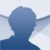# MathType

7.3.1.438

## By downloading MathType you will have a maths equation editor. You can create and export mathematical formulas to applications like Office or MATLAB

The vast majority of text processors have options that allow you to write and edit all kinds of formulas, but these tools usually lack functions that are really practical for those people that need a comfortable environment with which to develop math operations. Downloading MathType will offer you a wide range of resources to do so.

### The most comfortable environment to write maths formulas

MathType is an equation editor developed exclusively to carry out maths operations, that can use different technologies to recognize handwritten formulas, hotkeys or shortcuts. Its interface is ideal for the kind of application that we're dealing with, because it has a toolbar from which we'll be able to access almost any existing mathematical symbol and introduce it in our equation with a single click.

The most complete tool for the edition and writing of mathematical formulas.

### Features

• Mathematical equation and formula editor.
• Functions applicable to algebra, statistics, trigonometry, geometry, matrices and sets.
• Compatibility with other text and formulation edition applications.
• Total configuration of styles and formats.

### Compatible with other platforms

MathType stands out for its versatility. Once we've developed the mathematical problem that we want, we'll be able to export it so as to be able to use it in another application like Office, MATLAB, InDesign or Maple, among many others.

If you're looking for a complete and stable equation editor, downloading MathType is possibly one of the best alternatives available.